﻿ 基于柴-柴联合推进系统的动态特性仿真
 舰船科学技术2020, Vol. 42Issue (2): 115-120PDF

Simulation research on dynamic state characteristics of CODAD propulsion system
LIU Qi, LIU Yun-sheng, CHEN Xin-zhuan, WANG Meng, WU Yue
No. 92942 Unit of PLA, Beijing 100161, China
Abstract: In order to clarify the effect of parameter matching between the ship, the diesel engine and the propeller on the dynamic characteristics of ship propulsion system and provide a theoretical basis for the overall optimization and improvement in the later stage, the mathematical models of the subsystems such as diesel engine, gear box, shaft system and pitch propeller were established. The network hierarchy of propulsion system was built by using Simulink core components and the simulation calculation was carried out. The dynamic acceleration and deceleration characteristics of the propulsion system under the cold and heat engine conditions were analyzed and the simulation optimization were carried out. The results show that the speed of ship changes smoothly under the condition of heat engine deceleration, cold engine acceleration and cold engine deceleration, and the diesel engine is in ideal running condition. Under the accelerated condition of heat engine, the speed oscillation adjustment exists when the acceleration reaches the maximum, which easily leads to overload phenomenon of the diesel engine. This problem can be avoided by reasonably matching the adjustment time of the acceleration process, and the dynamic characteristics of propulsion system can be optimized.
Key words: CODAD     propulsion system     dynamic characteristics     ship speed
0 引　言

1 推进系统数学模型 1.1 柴油机

 $\frac{{{\text{π}} {I_e}}}{{30}}\frac{{{\rm d}{N_d}}}{{dt}} = {M_i} - {M_B} - {M_f} {\text{。}}$ (1)

 ${M_i} = {{{H_u}{g_c}{\eta _i}} / {\tau{\text{π}} }} {\text{，}}$ (2)
 ${M_e} = {M_i} \cdot {\eta _m} {\text{。}}$ (3)

 ${\eta _i} = ({C_1} + {C_2}{N_d} - {C_3}N_d^3)(1 - {C_4}{\alpha ^{ - {C_5}}}) {\text{，}}$ (4)

 ${\eta _m} = \frac{{{p_e}}}{{{p_i}}} = \frac{{{p_e}}}{{{p_e} + {p_m}}} {\text{。}}$ (5)

1.2 齿轮箱

 ${N_d} = {N_p} \cdot i {\text{，}}$ (6)
 ${Q_d} = {Q_p} \cdot i {\text{，}}$ (7)
 ${I_d} = {I_p} \cdot i {\text{。}}$ (8)

1.3 轴系

 ${M_e} \cdot i \cdot T \cdot {\eta _{gb}} - {M_{f2}} - {M_p} = {I_s} \cdot \frac{{\text{π}} }{{30}} \cdot \frac{{{\text{d}}{N_p}}}{{{\text{d}}t}} {\text{。}}$ (9)

 ${M_{f2}} = \left( {\frac{1}{3} + \frac{2}{3} \cdot \frac{{{N_p}}}{{{N_{d0}}}} \cdot i} \right){M_{fd0}} {\text{。}}$ (10)

 ${I_s} = {I_1} \cdot {i^2} \cdot {\eta _{gb}} + {I_2} + {I_3} {\text{。}}$ (11)

1.4 调距桨

 ${T_p} = {K_T}\rho N_p^2{D^4} {\text{，}}$ (12)
 ${M_p} = {K_Q}\rho N_p^2{D^5} {\text{。}}$ (13)

 ${T_e} = {T_p}\left( {1 - {t_p}t} \right) {\text{，}}$ (14)
 ${V_p} = {V_s}\left( {1 - w} \right) {\text{。}}$ (15)

 ${t_p} = \left\{ {\begin{array}{*{20}{c}} 3 {\text{，}}&{P/D \leqslant {\rm{ - 1}}} {\text{，}} \\ { - 3P/D} {\text{，}}&{{\rm{ - 1 < }}P/D{\rm{ < 0}}} {\text{，}} \\ {{\rm{ }}P/D} {\text{，}}&{{\rm{0}} \leqslant {\rm{ }}P/D{\rm{ < 1}}} {\text{，}}\\ 1 {\text{，}}&{P/D \geqslant {\rm{1}}} {\text{。}} \end{array}} \right.$ (16)
2 仿真模型及参数设置表 1 动态控制参数 Tab.1 Dynamic control parameters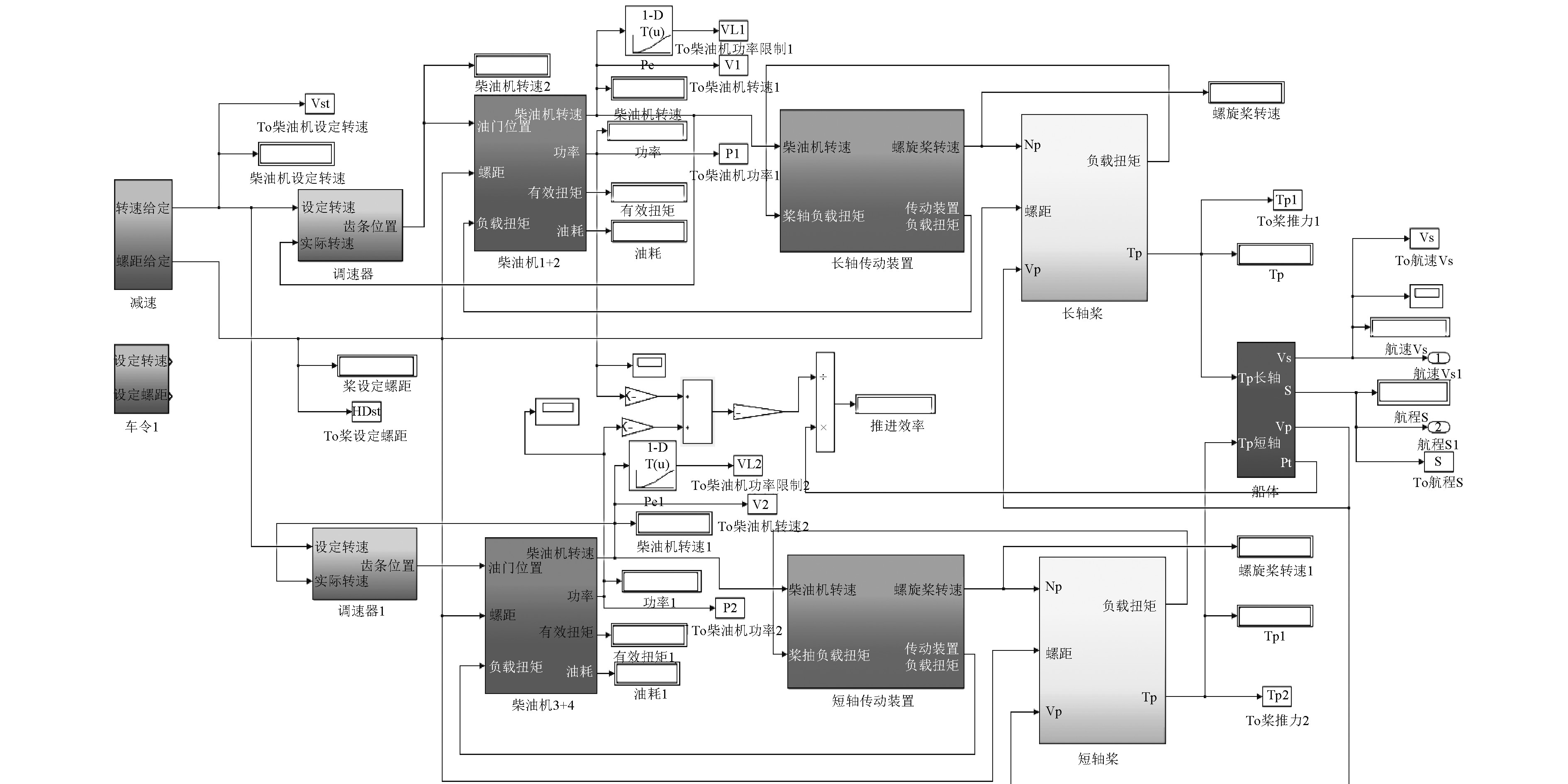图 1 推进系统仿真模型 Fig. 1 Propulsion system simulation model
3 结果分析 3.1 加速工况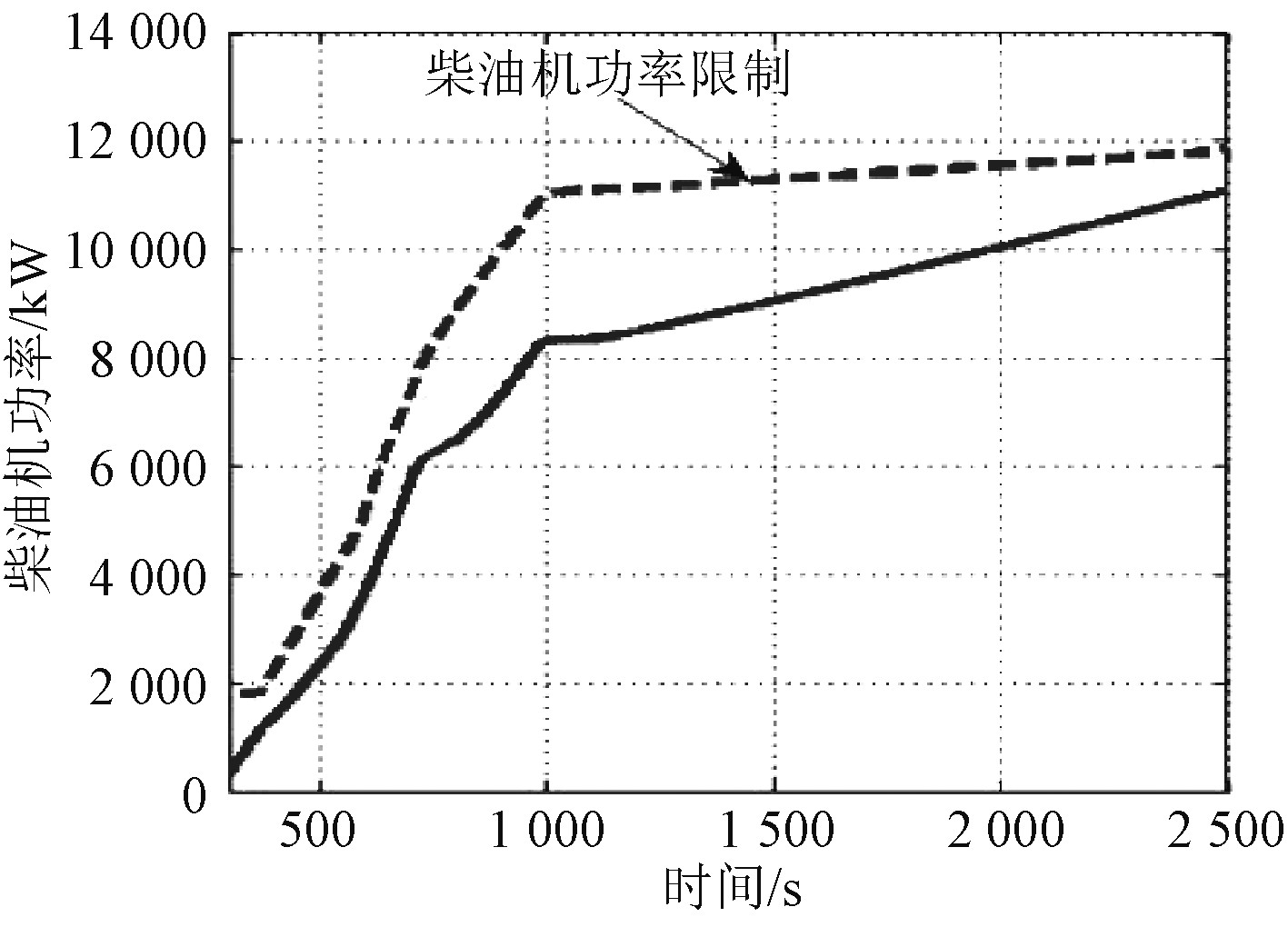图 2 冷机正常加速过程主机功率变化规律 Fig. 2 Power variation of main engine during normal acceleration of cooling engine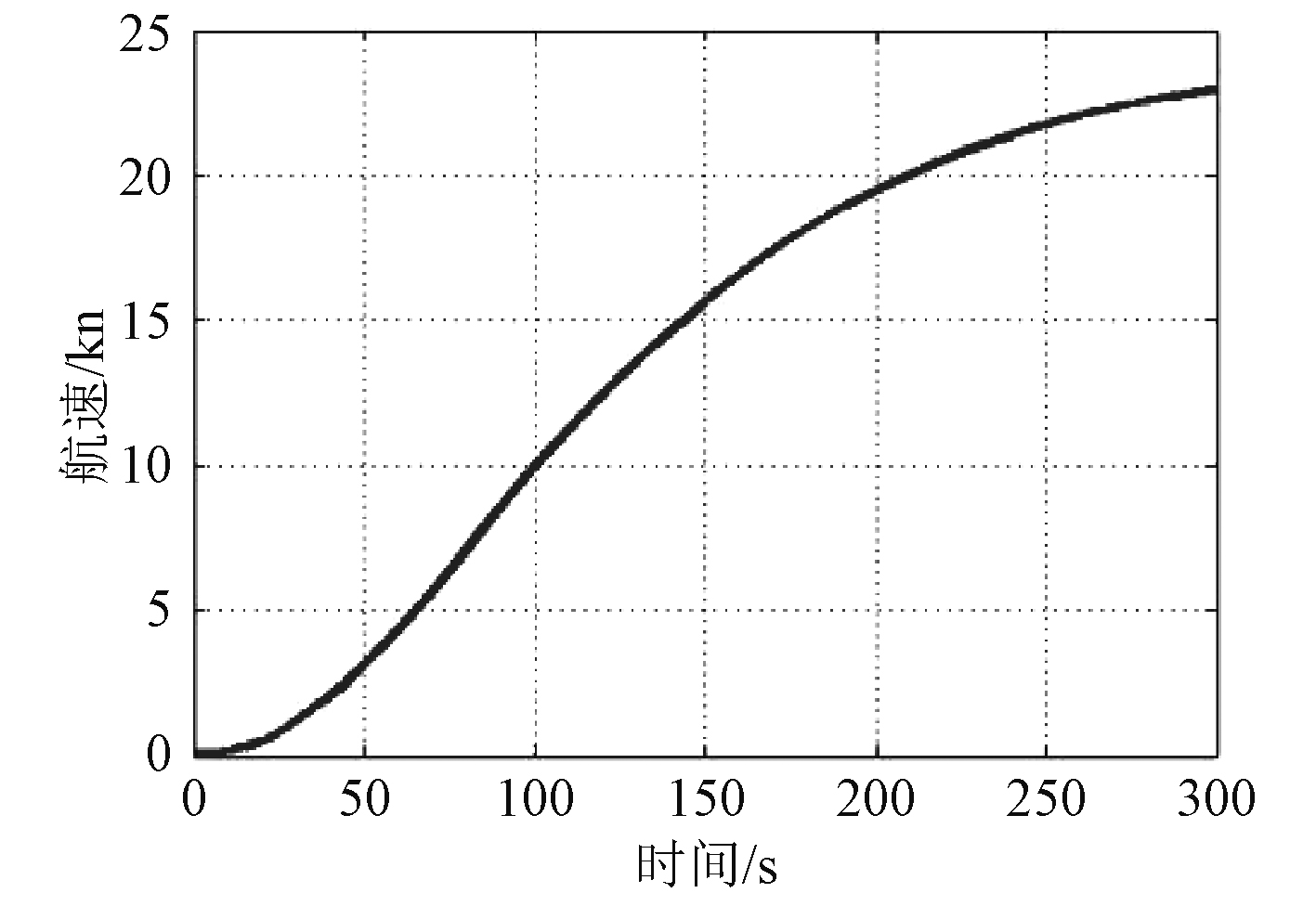图 9 热机应急加速过程航速变化规律 Fig. 9 Ship speed variation during emergency acceleration of heat engine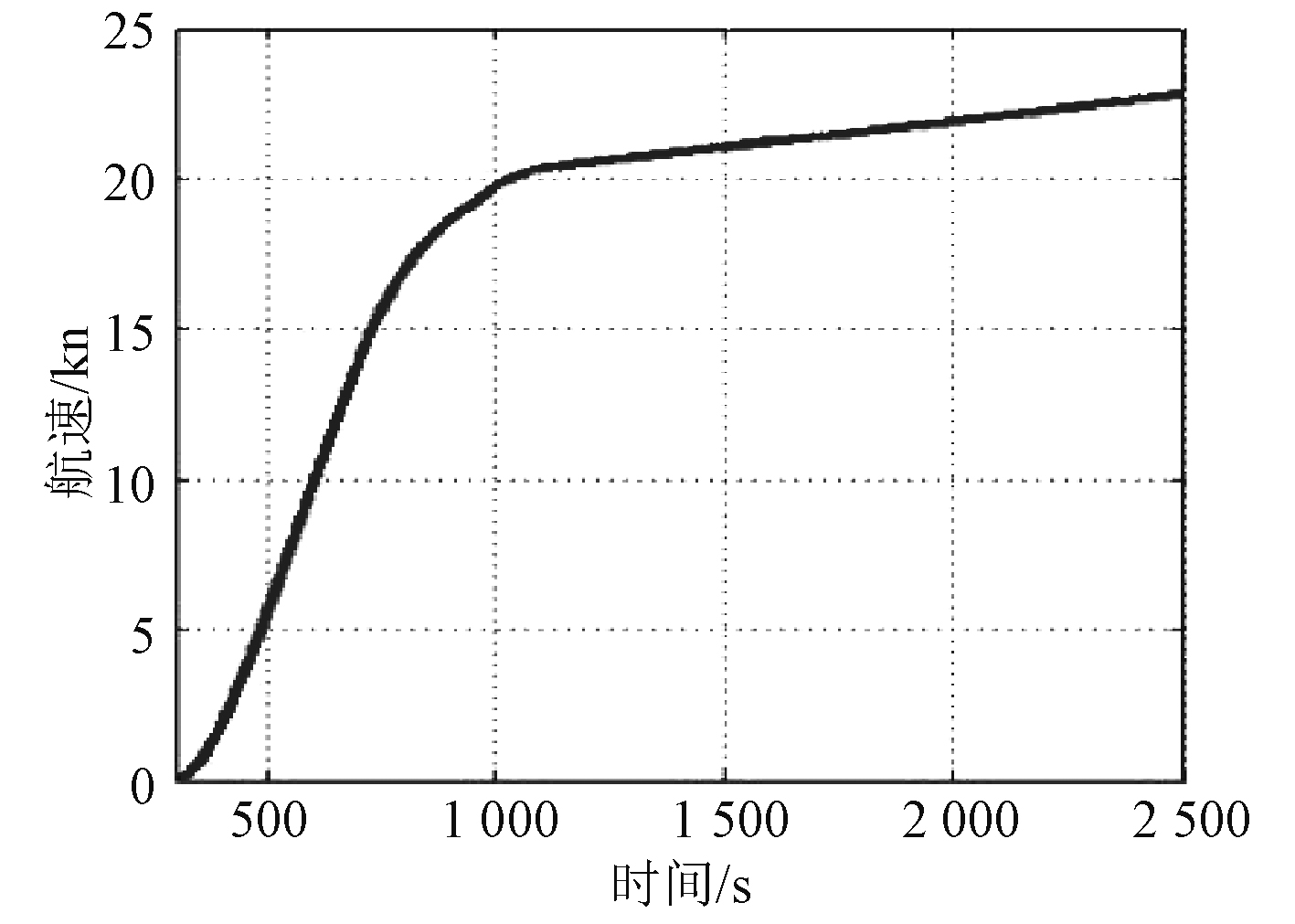图 3 冷机正常加速过程航速变化规律 Fig. 3 Ship speed variation during normal acceleration of cooling engine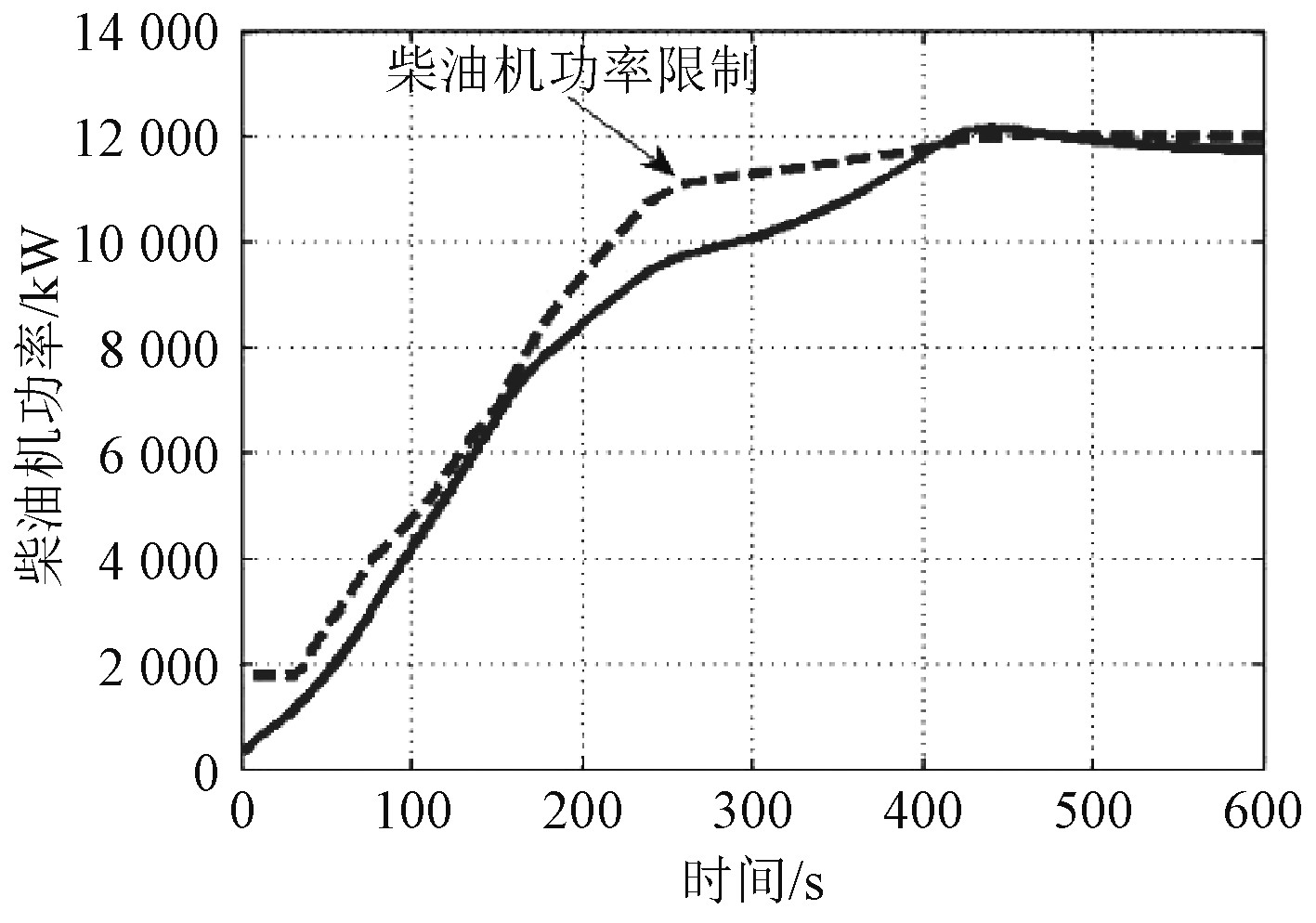图 4 冷机应急加速过程主机功率变化规律 Fig. 4 Power variation of main engine during emergency acceleration of cooling engine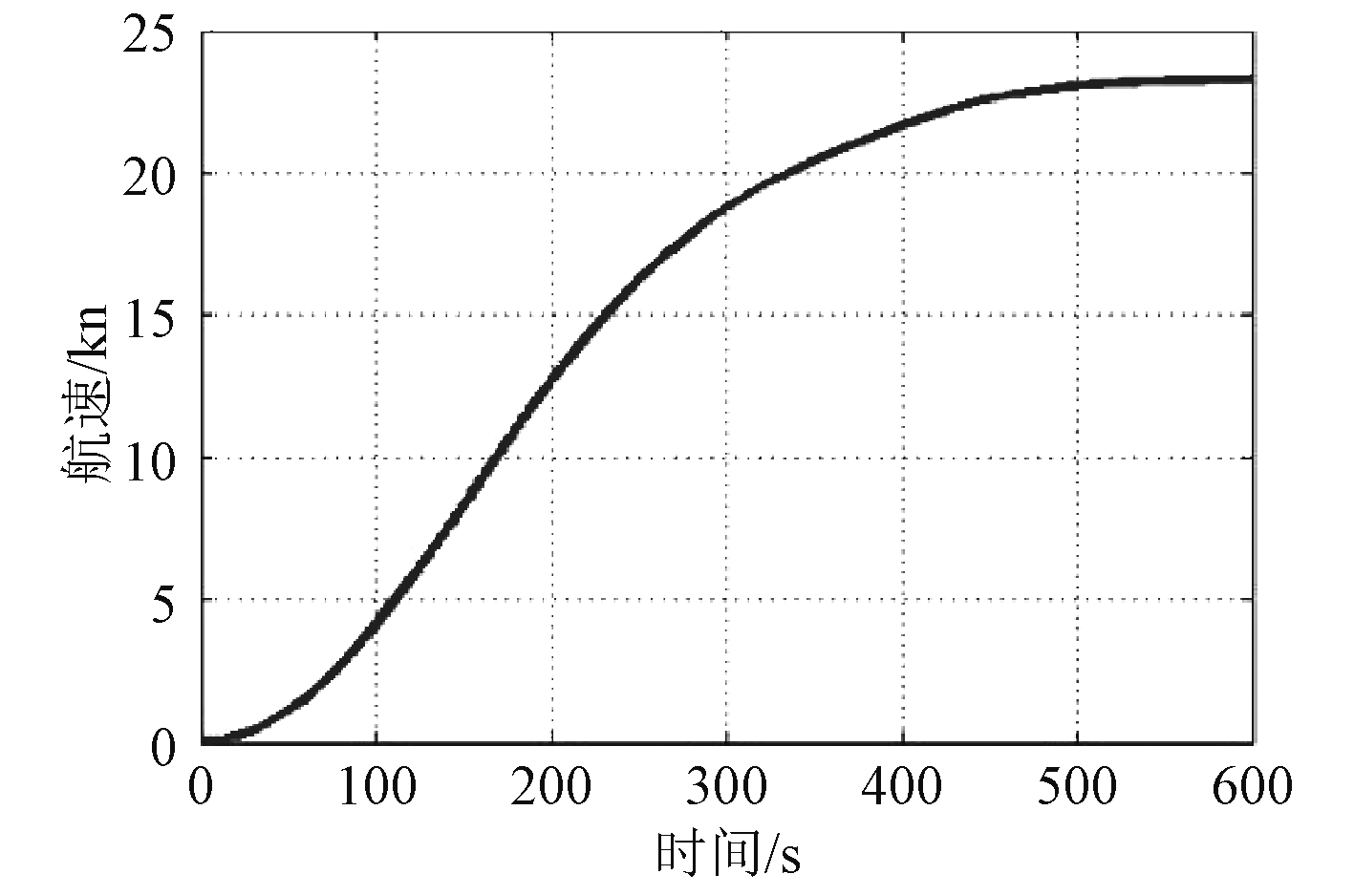图 5 冷机应急加速过程航速变化规律 Fig. 5 Ship speed variation during emergency acceleration of cooling engine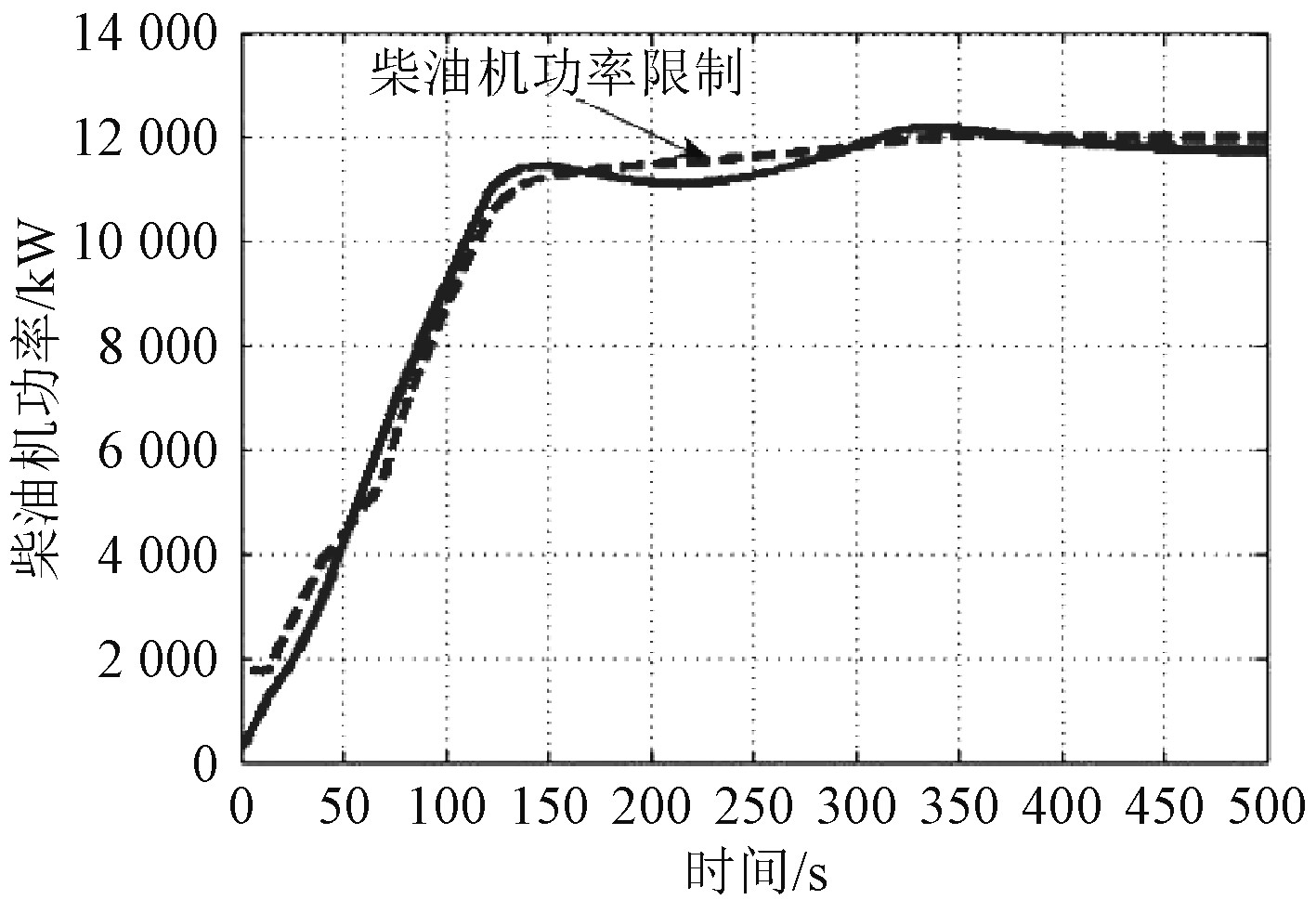图 6 热机正常加速过程主机功率变化规律 Fig. 6 Power variation of main engine during normal acceleration of heat engine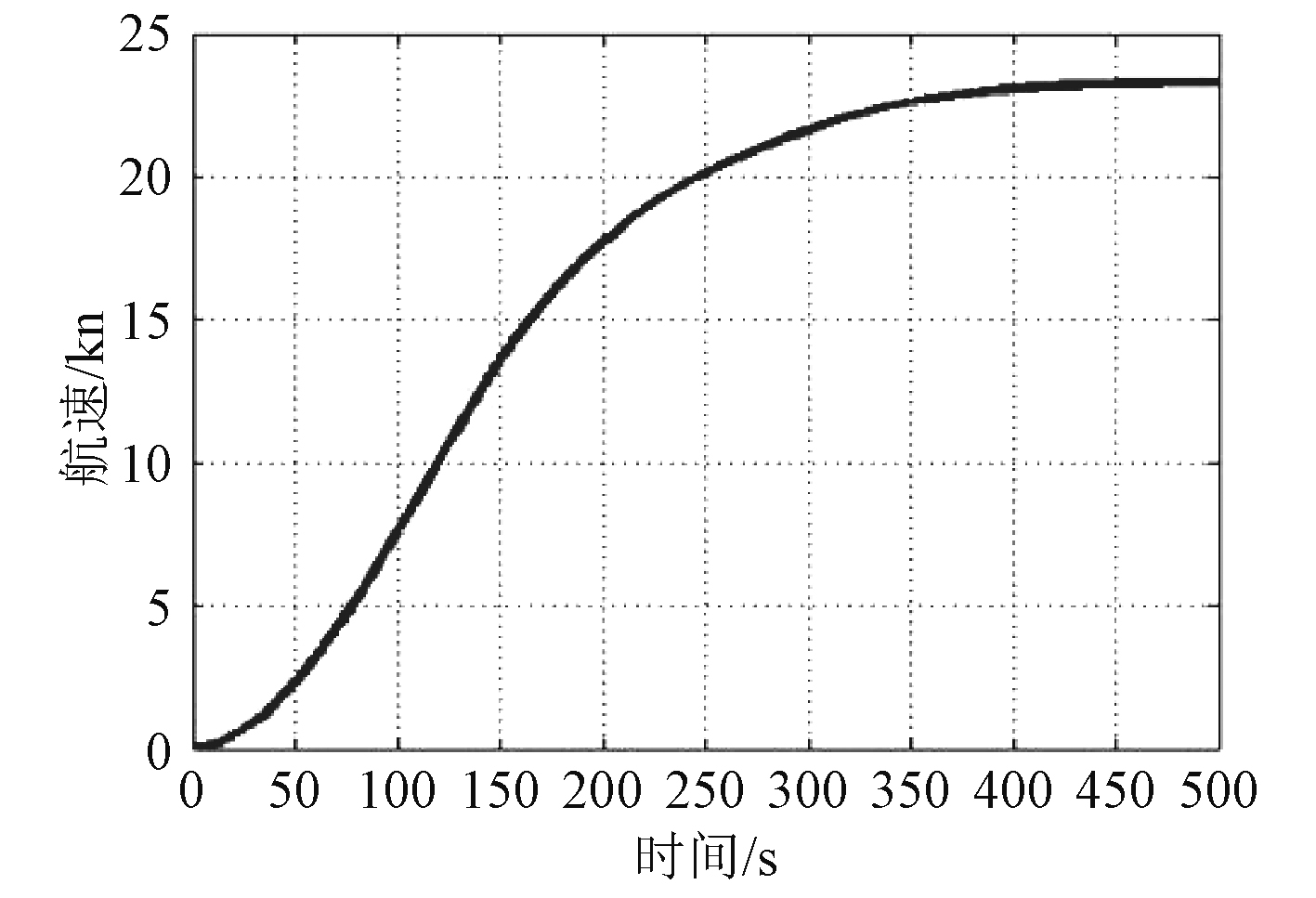图 7 热机正常加速过程航速变化规律 Fig. 7 Ship speed variation during normal acceleration of heat engine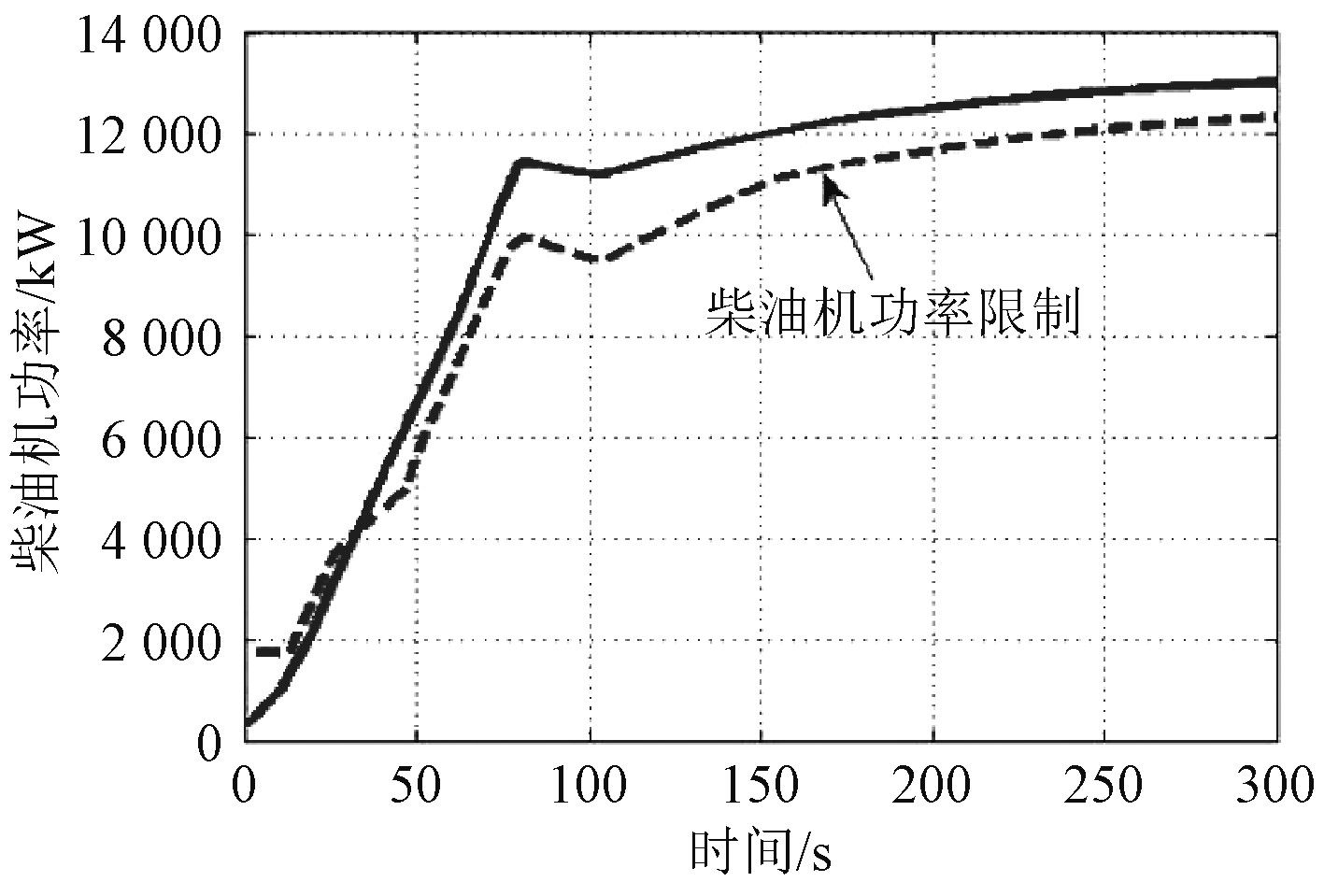图 8 热机应急加速过程主机功率变化规律 Fig. 8 Power variation of main engine during emergency acceleration of heat engine
3.2 减速工况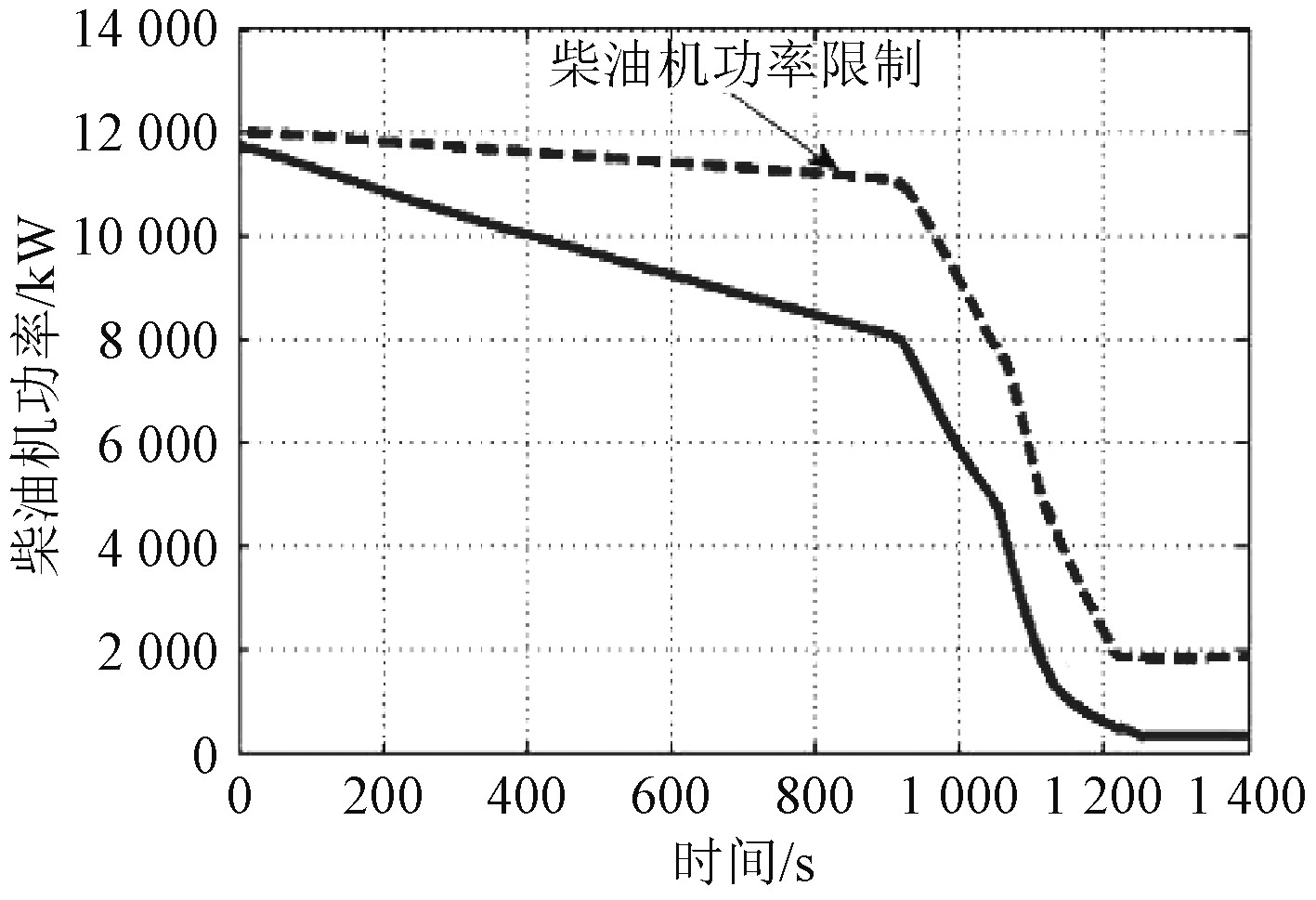图 10 冷机正常减速过程主机功率变化规律 Fig. 10 Power variation of main engine during normal deceleration of cooling engine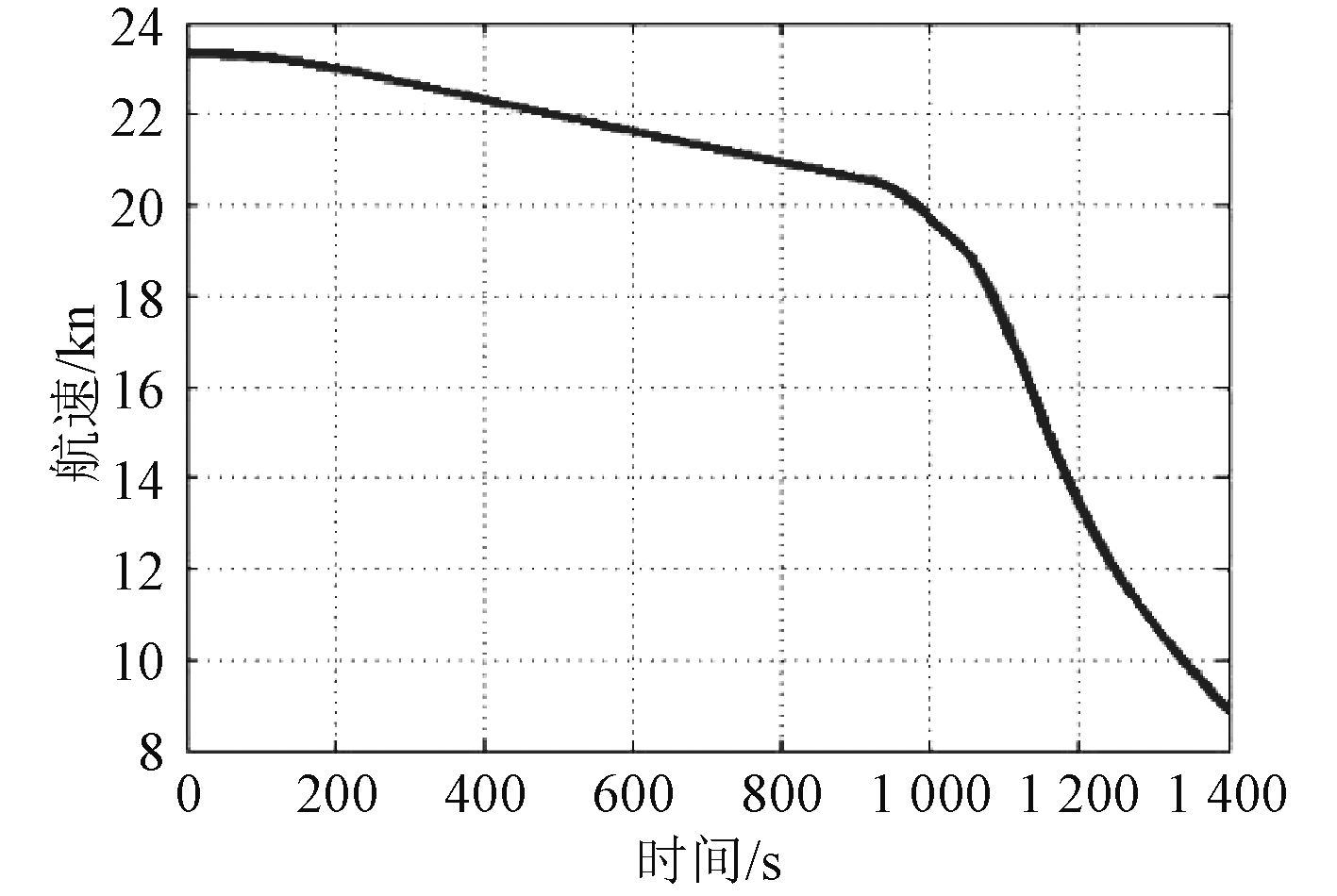图 11 冷机正常减速过程航速变化规律 Fig. 11 Ship speed variation during normal deceleration of cooling engine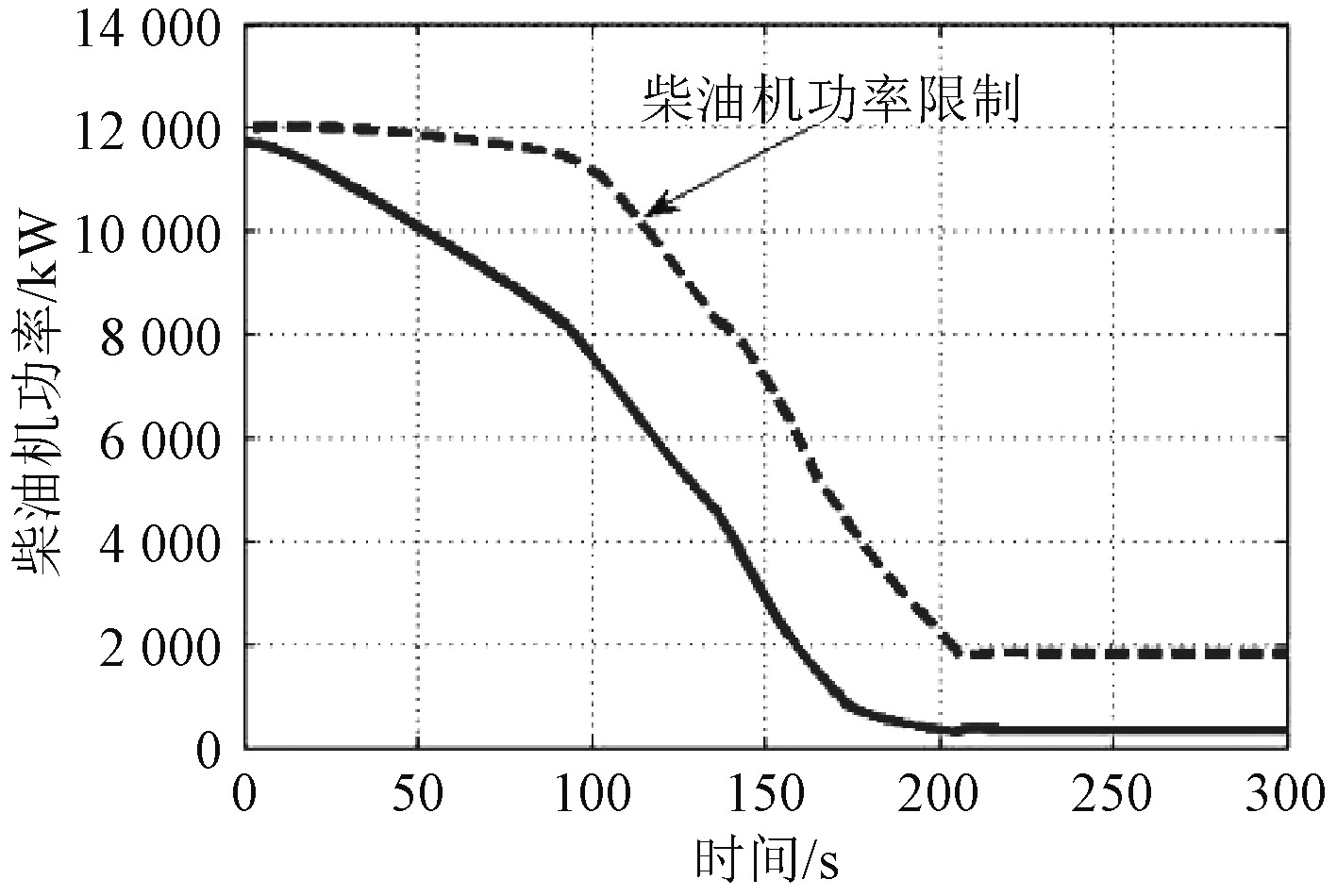图 12 冷机应急减速过程主机功率变化规律 Fig. 12 Power variation of main engine during emergency deceleration of cooling engine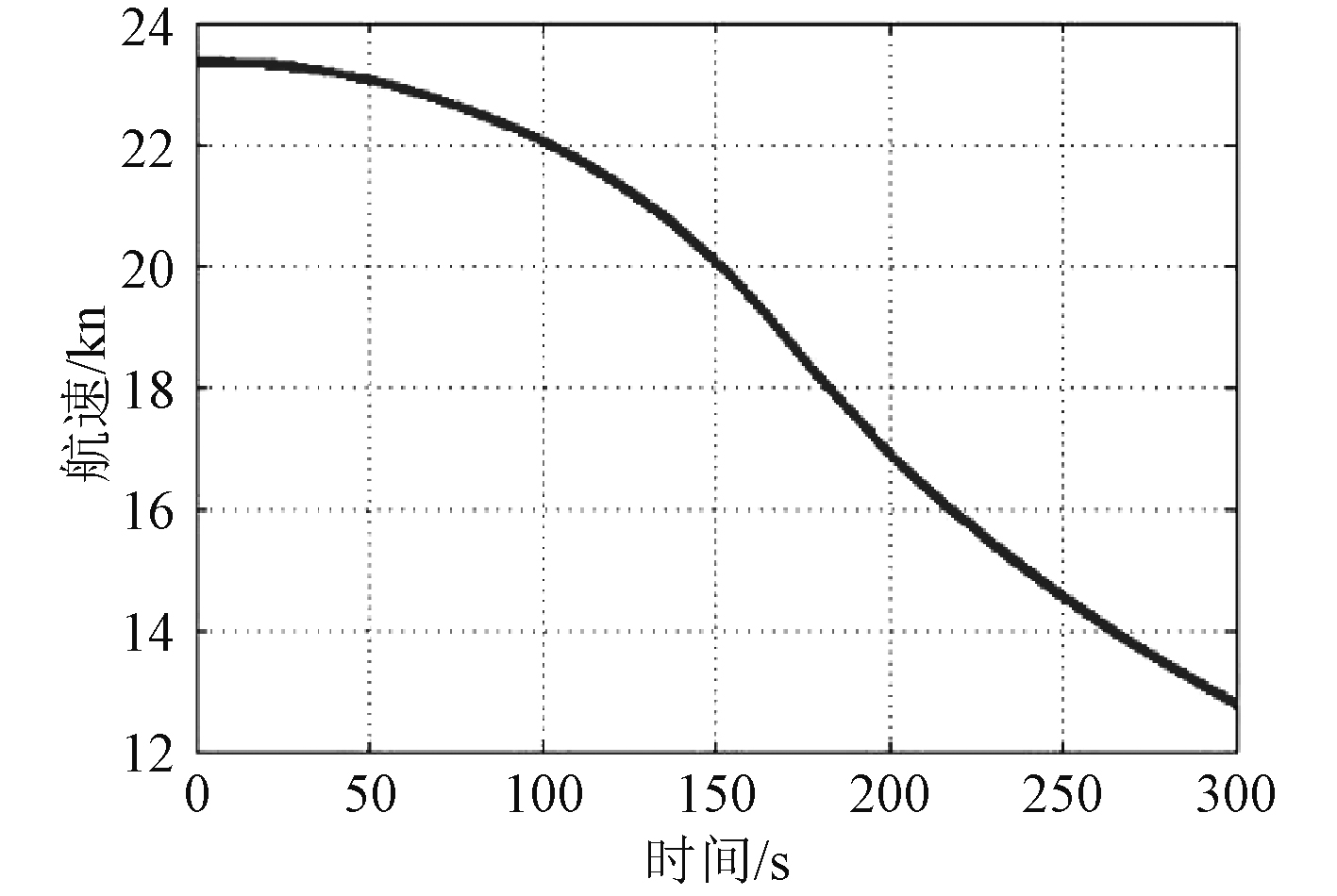图 13 冷机应急减速过程航速变化规律 Fig. 13 Ship speed variation during emergency deceleration of cooling engine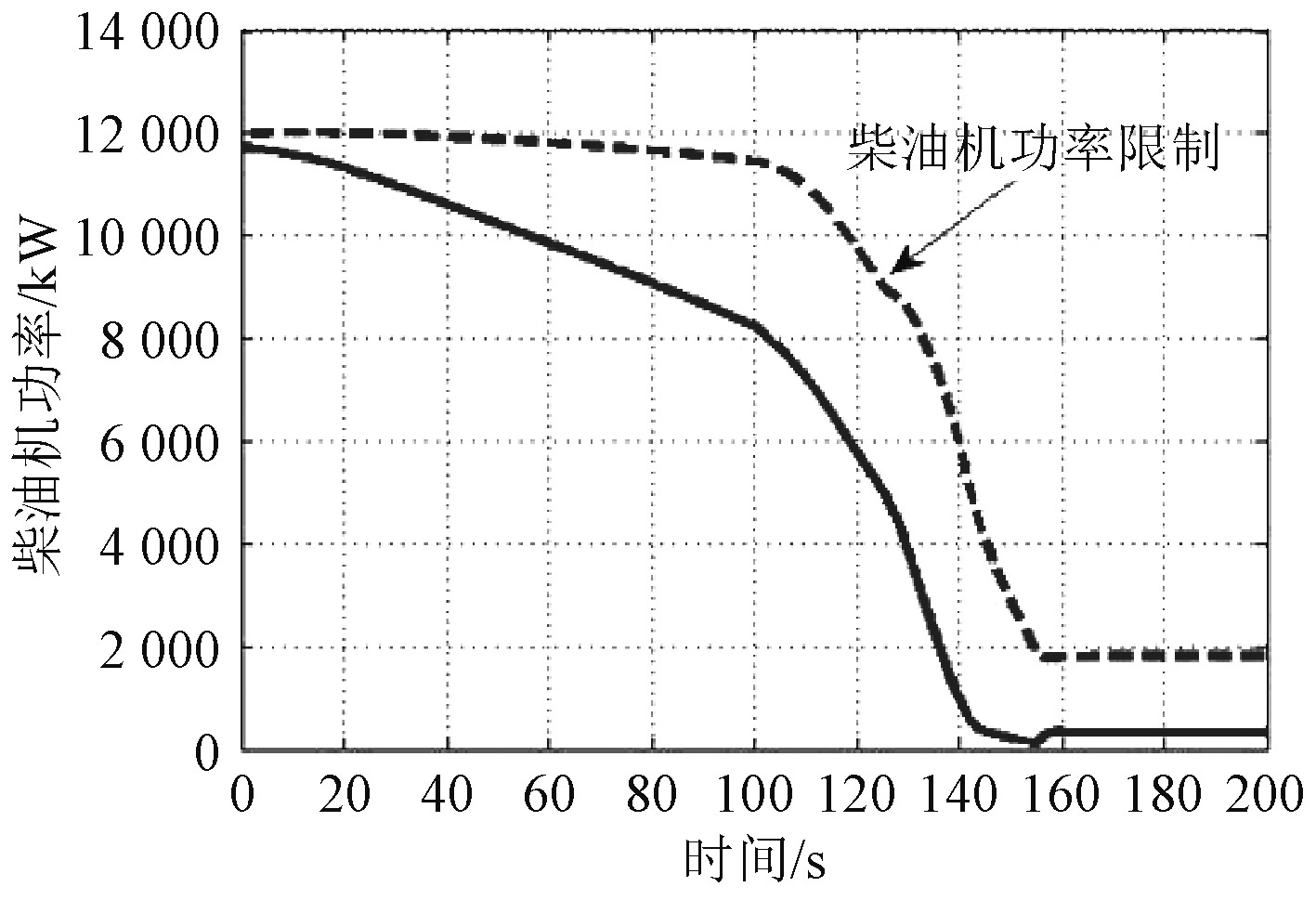图 14 热机正常减速过程主机功率变化规律 Fig. 14 Power variation of main engine during normal deceleration of heat engine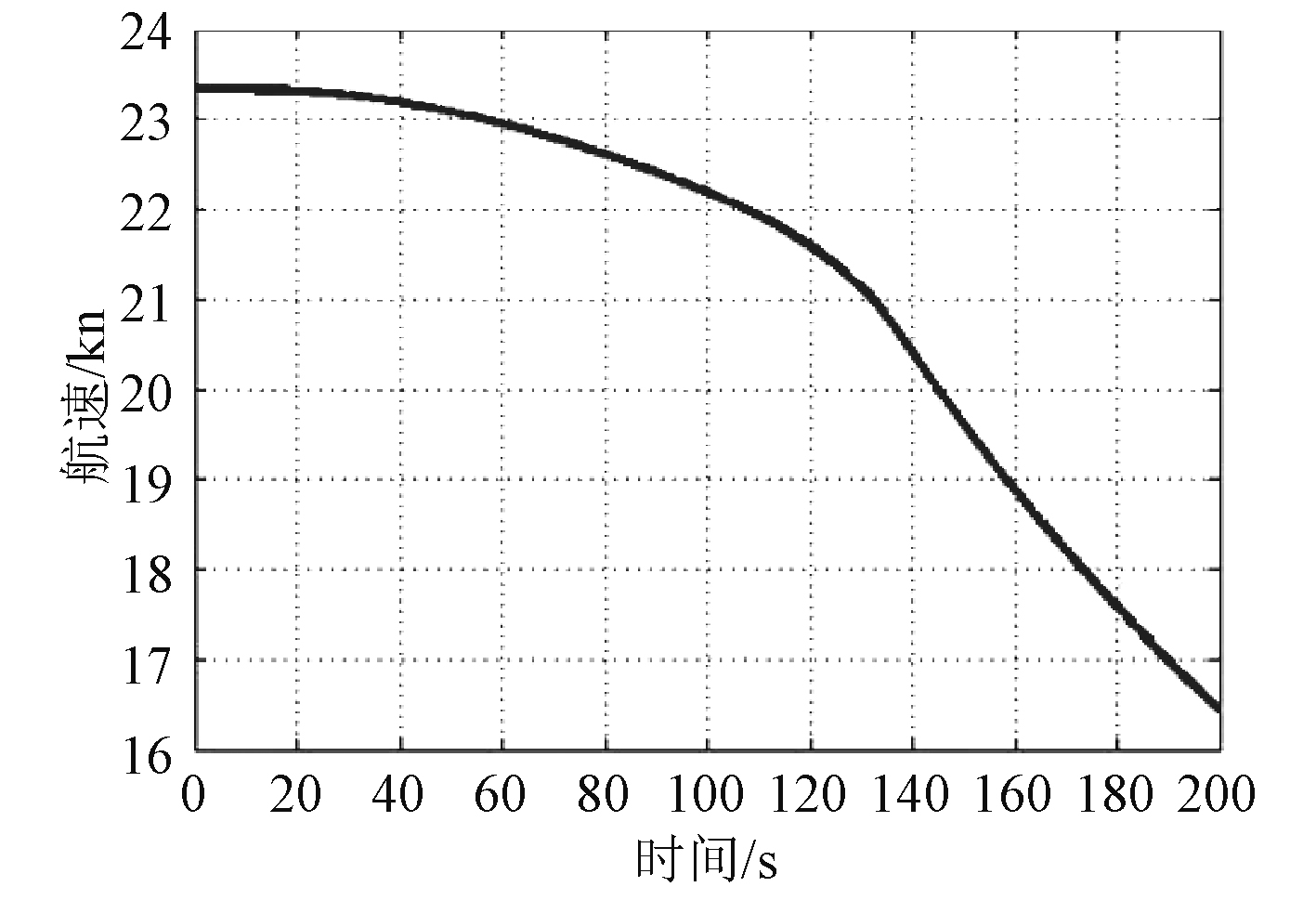图 15 热机正常减速过程航速变化规律 Fig. 15 Ship speed variation during normal deceleration of heat engine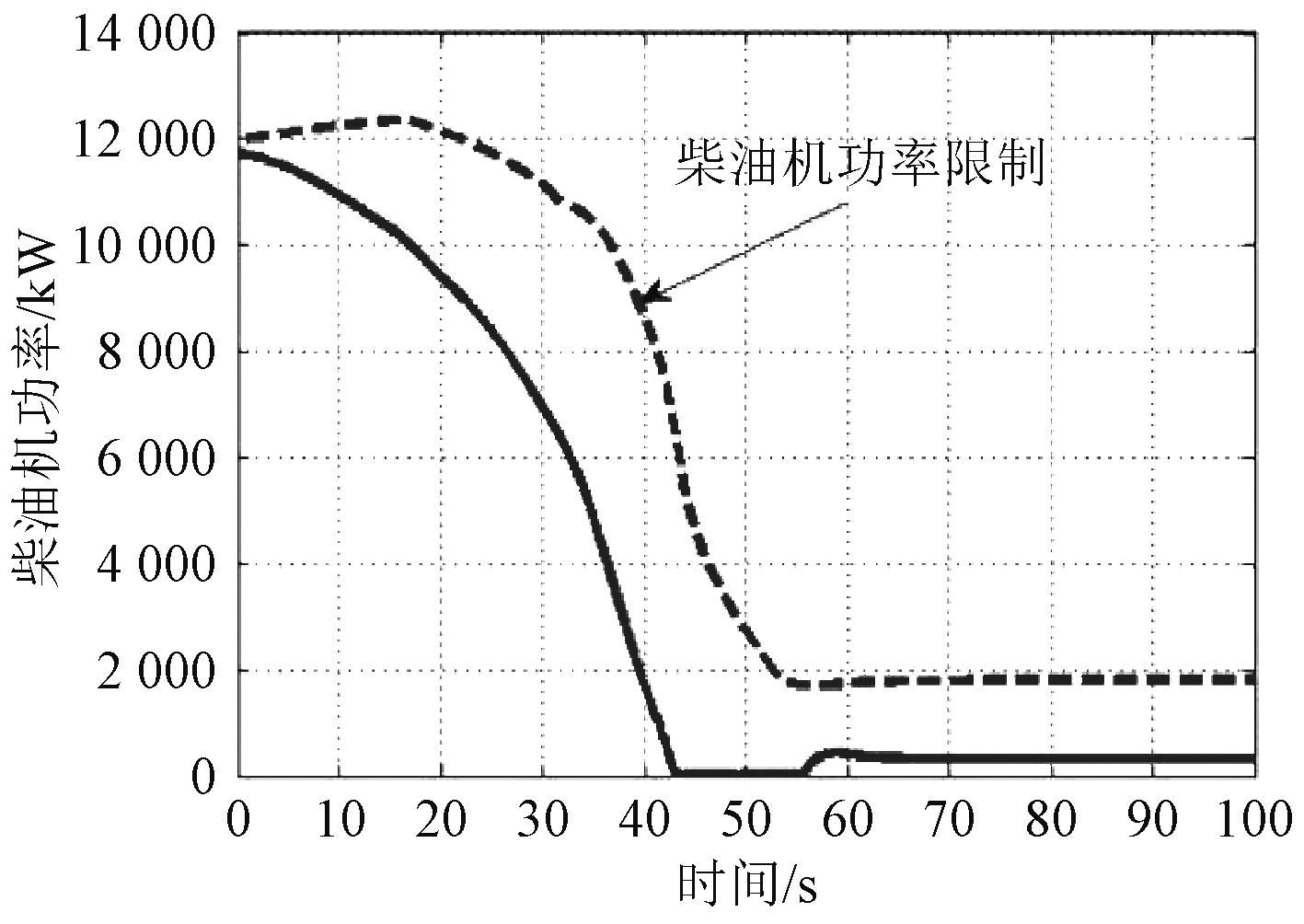图 16 热机应急减速过程主机功率变化规律 Fig. 16 Power variation of main engine during emergency deceleration of heat engine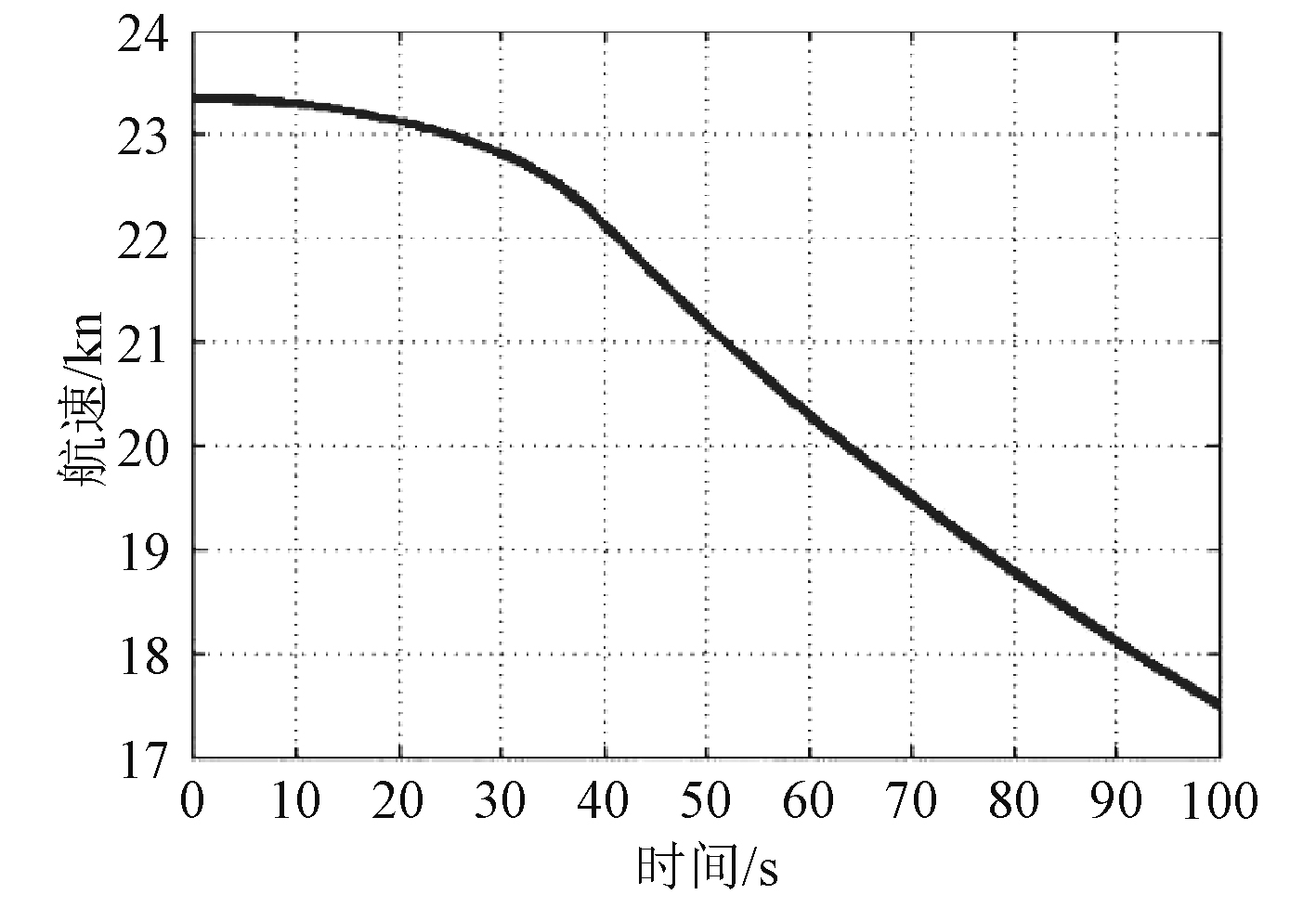图 17 热机应急减速过程航速变化规律 Fig. 17 Ship speed variation during emergency deceleration of heat engine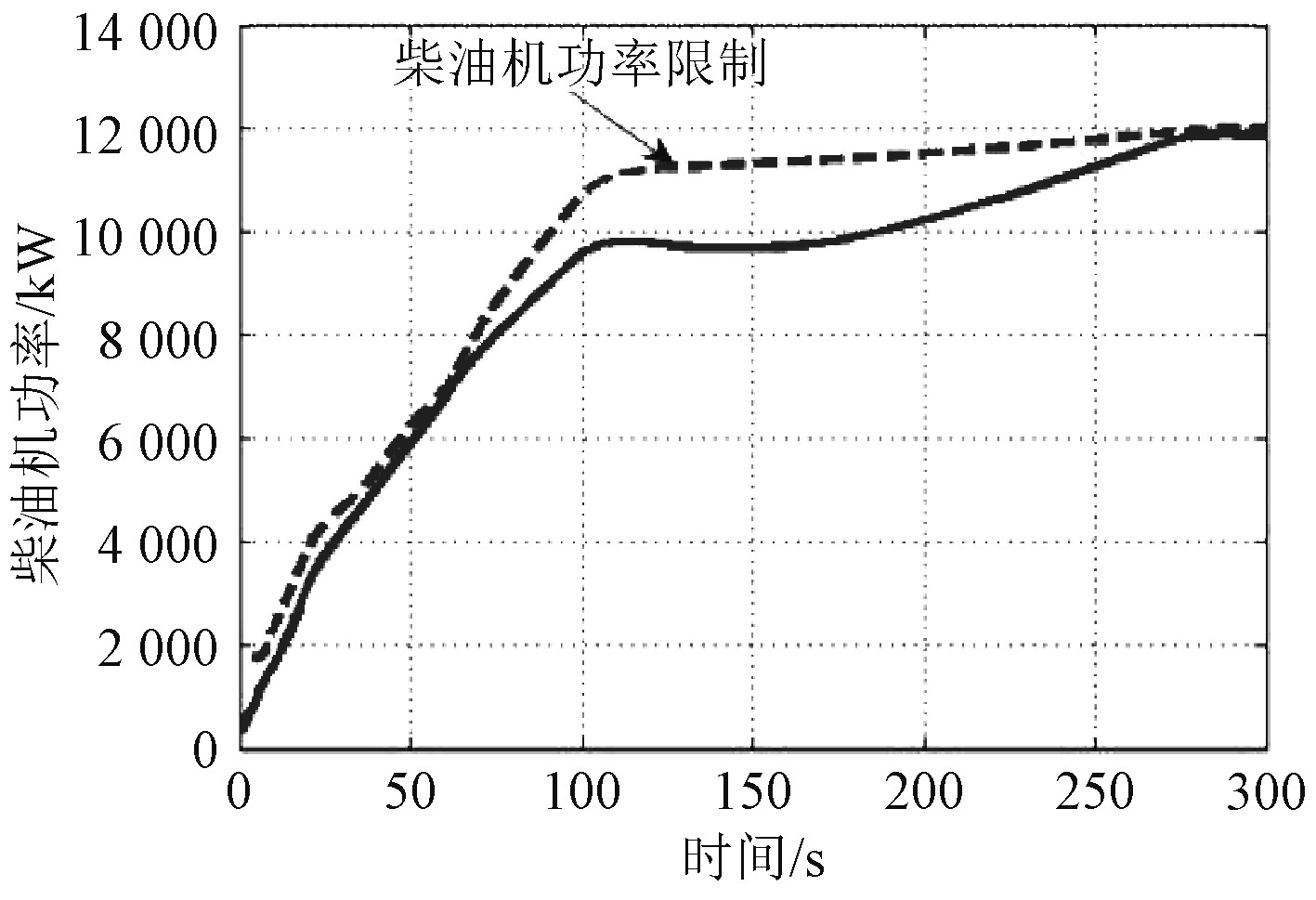图 18 热机应急加速过程优化后主机功率变化规律 Fig. 18 Power variation of main engine after optimization of heat engine emergency acceleration
3.3 热机应急加速工况优化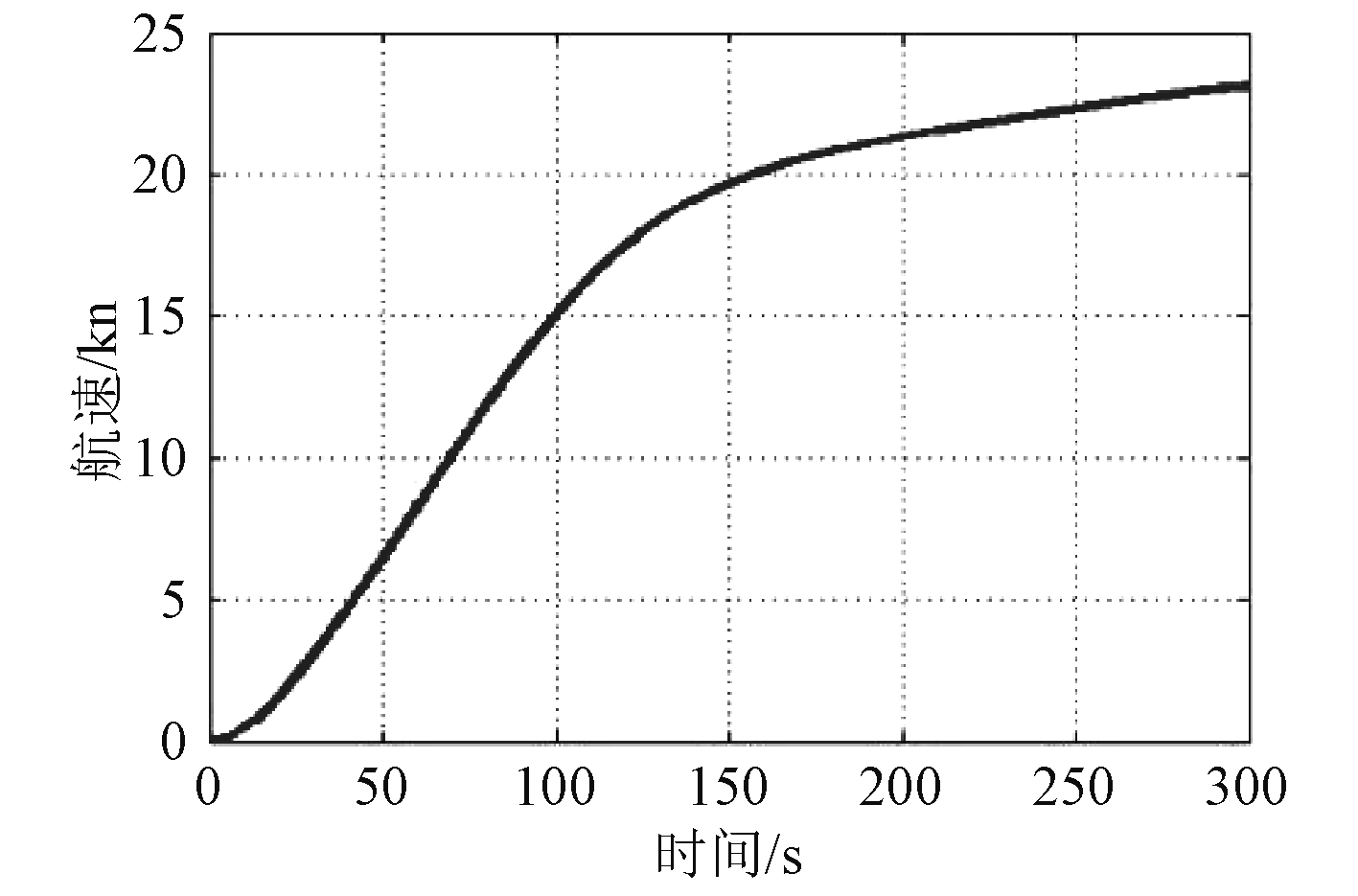图 19 热机应急加速过程优化后航速变化规律 Fig. 19 Ship speed variation after optimization of heat engine emergency acceleration
4 结　语